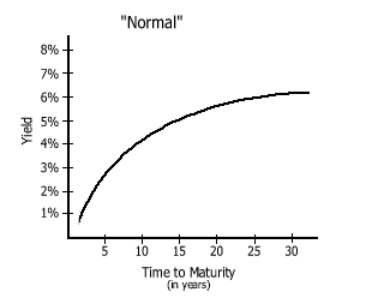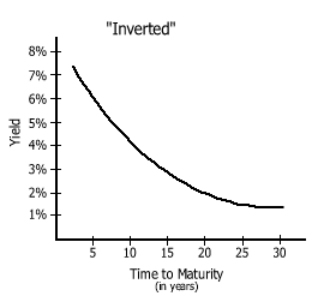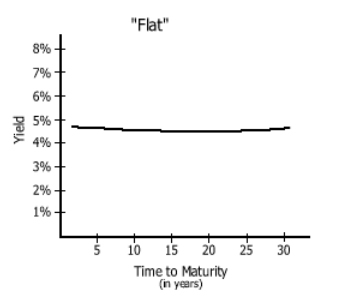# Term Structure of Interest Rates

Updated September 16, 2020

## What is the Term Structure of Interest Rates?

The term structure of interest rates, also called the yield curve, is a graph that plots the yields of similar-quality bonds against their maturities, from shortest to longest.

## How Does the Term Structure of Interest Rates Work?

The term structure of interest rates shows the various yields that are currently being offered on bonds of different maturities. It enables investors to quickly compare the yields offered on short-term, medium-term and long-term bonds.

Note that the chart does not plot coupon rates against a range of maturities -- that graph is called the spot curve.

The term structure of interest rates takes three primary shapes. If short-term yields are lower than long-term yields, the curve slopes upwards and the curve is called a positive (or "normal") yield curve. Below is an example of a normal yield curve:If short-term yields are higher than long-term yields, the curve slopes downwards and the curve is called a negative (or "inverted") yield curve. Below is example of an inverted yield curve:Finally, a flat term structure of interest rates exists when there is little or no variation between short and long-term yield rates. Below is an example of a flat yield curve:It is important that only bonds of similar risk are plotted on the same yield curve. The most common type of yield curve plots Treasury securities because they are considered risk-free and are thus a benchmark for determining the yield on other types of debt.

The shape of the curve changes over time. Investors who are able to predict how term structure of interest rates will change can invest accordingly and take advantage of the corresponding changes in bond prices.

Term structure of interest rates are calculated and published by The Wall Street Journal, the Federal Reserve, and a variety of other financial institutions.

## Why Does the Term Structure of Interest Rates Matter?

In general, when the term structure of interest rates curve is positive, this indicates that investors desire a higher rate of return for taking the increased risk of lending their money for a longer time period.

Many economists also believe that a steep positive curve means that investors expect strong future economic growth with higher future inflation (and thus higher interest rates), and that a sharply inverted curve means that investors expect sluggish economic growth with lower future inflation (and thus lower interest rates). A flat curve generally indicates that investors are unsure about future economic growth and inflation.

There are three central theories that attempt to explain why yield curves are shaped the way they are.

1. The "expectations theory" says that expectations of increasing short-term interest rates are what create a normal curve (and vice versa).

2. The "liquidity preference hypothesis" says that investors always prefer the higher liquidity of short-term debt and therefore any deviance from a normal curve will only prove to be a temporary phenomenon.

3. The "segmented market hypothesis" says that different investors adhere to specific maturity segments. This means that the term structure of interest rates is a reflection of prevailing investment policies.

Because the term structure of interest rates is generally indicative of future interest rates, which are indicative of an economy's expansion or contraction, yield curves and changes in these curves can provide a great deal of information. In the 1990s, Duke University professor Campbell Harvey found that inverted yield curves have preceded the last five U.S. recessions.

Changes in the shape of the term structure of interest rates can also have an impact on portfolio returns by making some bonds relatively more or less valuable compared to other bonds. These concepts are part of what motivate analysts and investors to study the term structure of interest rates carefully.